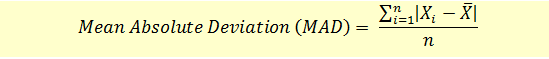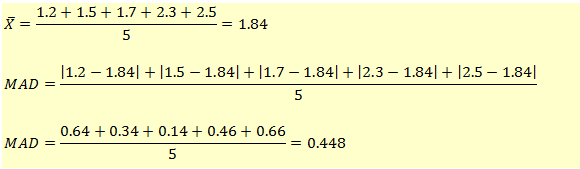# Range and Mean Absolute Deviation

In investment management, one of the most important things for an investor is the trade-off between the returns and risk from an investment. The return or reward is measured using the measures of central tendency while the risk is measured using the measures of dispersion. The dispersion here refers to how the observations vary around the mean.

We will now look at the different types of measures of dispersion.

### Range

Range is calculated as the difference between the highest value and the lowest value.

Range = Highest Value – Lowest Value

Refer to our data set:

1.2, 1.5, 1.7, 2.3, 2.5, 2.9, 3, 3.8, 4.2, 4.3, 5.4, 5.5, 5.6, 5.9, 6.2, 6.7, 8.5, 8.8, 9.5, 9.8

The lowest value is 1.2 and the highest value is 9.8

The range will be given as:

Range = 9.8 – 1.2 = 8.6

### Mean Absolute Deviation

Mean absolute deviation is calculated as the average of the absolute values of difference between each observation and the arithmetic mean.Note that we take the absolute value of differences. So, any negative signs are ignored.

Let’s take the following data set of five values:

1.2, 1.5, 1.7, 2.3, 2.5Both Range and Mean Absolute Deviation are not very commonly used measures are dispersion. We will now look at the more robust measures, namely, variance and standard deviation.

Get our Data Science for Finance Bundle for just $29$51.